# Chapter 6 - Electronic Spectroscopy of Molecules.pdf

12 de Apr de 2023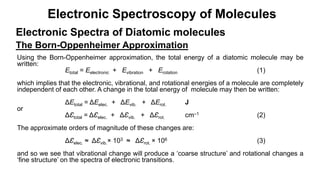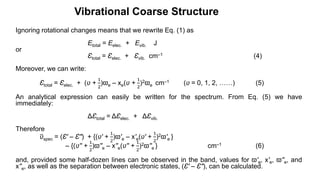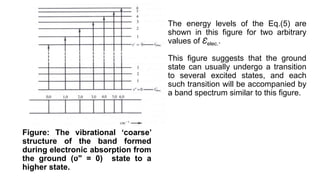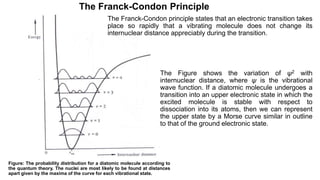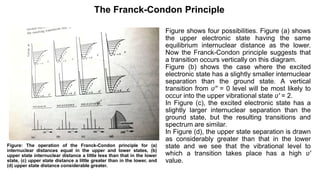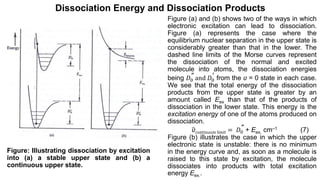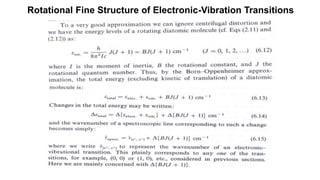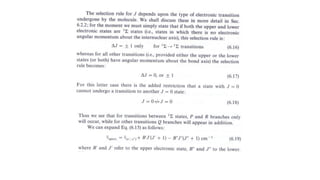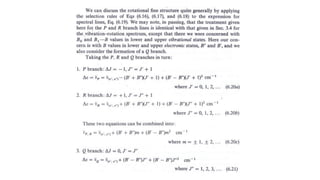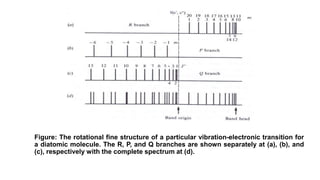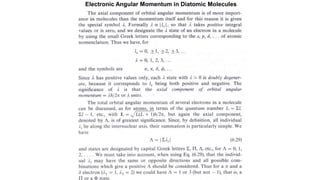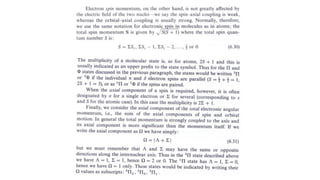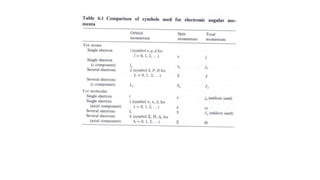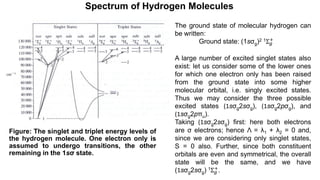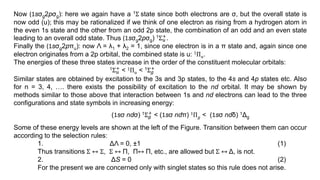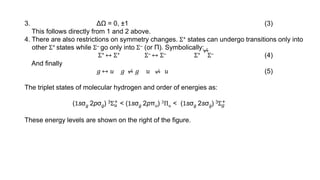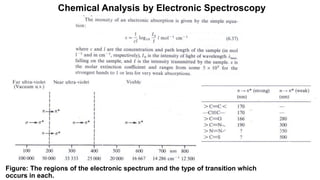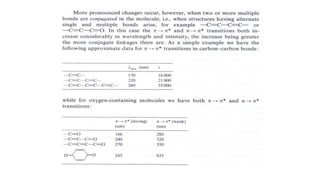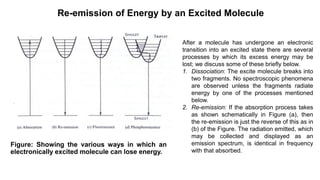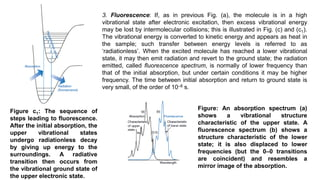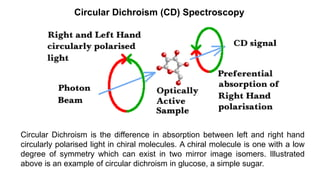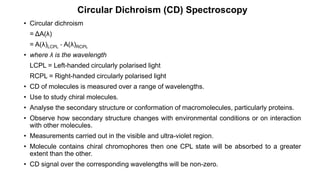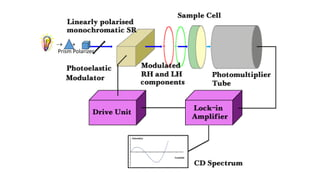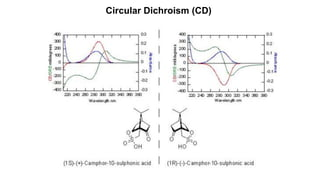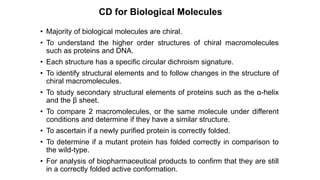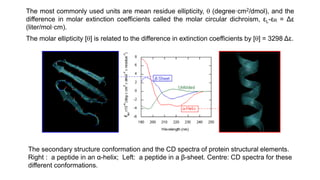1 de 27

### Chapter 6 - Electronic Spectroscopy of Molecules.pdf

• 1. Electronic Spectroscopy of Molecules Electronic Spectra of Diatomic molecules The Born-Oppenheimer Approximation Using the Born-Oppenheimer approximation, the total energy of a diatomic molecule may be written: Etotal = Eelectronic + Evibration + Erotation (1) which implies that the electronic, vibrational, and rotational energies of a molecule are completely independent of each other. A change in the total energy of molecule may then be written: ΔEtotal = ΔEelec. + ΔEvib. + ΔErot. J or ΔƐtotal = ΔƐelec. + ΔƐvib. + ΔƐrot. cm‒1 (2) The approximate orders of magnitude of these changes are: ΔƐelec. ≈ ΔƐvib.× 103 ≈ ΔƐrot. × 106 (3) and so we see that vibrational change will produce a ‘coarse structure’ and rotational changes a ‘fine structure’ on the spectra of electronic transitions.
• 2. Vibrational Coarse Structure Ignoring rotational changes means that we rewrite Eq. (1) as Etotal = Eelec. + Evib. J or Ɛtotal = Ɛelec. + Ɛvib. cm‒1 (4) Moreover, we can write: Ɛtotal = Ɛelec. + (ʋ + 1 2 )ϖe ‒ xe(ʋ + 1 2 )2ϖe cm‒1 (ʋ = 0, 1, 2, ……) (5) An analytical expression can easily be written for the spectrum. From Eq. (5) we have immediately: ΔƐtotal = ΔƐelec. + ΔƐvib. Therefore ʋspec = (Ɛ' – Ɛʺ) + {(ʋ' + 1 2 )ϖ'e ‒ x'e(ʋ' + 1 2 )2ϖ'e } ‒ {(ʋ'' + 1 2 )ϖ''e ‒ x''e(ʋ'' + 1 2 )2ϖ''e } cm‒1 (6) and, provided some half-dozen lines can be observed in the band, values for ϖ'e, x'e, ϖ''e, and x''e, as well as the separation between electronic states, (Ɛ' – Ɛʺ), can be calculated.
• 3. Figure: The vibrational ‘coarse’ structure of the band formed during electronic absorption from the ground (ʋʺ = 0) state to a higher state. The energy levels of the Eq.(5) are shown in this figure for two arbitrary values of Ɛelec.. This figure suggests that the ground state can usually undergo a transition to several excited states, and each such transition will be accompanied by a band spectrum similar to this figure.
• 4. The Franck-Condon Principle The Franck-Condon principle states that an electronic transition takes place so rapidly that a vibrating molecule does not change its internuclear distance appreciably during the transition. Figure: The probability distribution for a diatomic molecule according to the quantum theory. The nuclei are most likely to be found at distances apart given by the maxima of the curve for each vibrational state. The Figure shows the variation of ψ2 with internuclear distance, where ψ is the vibrational wave function. If a diatomic molecule undergoes a transition into an upper electronic state in which the excited molecule is stable with respect to dissociation into its atoms, then we can represent the upper state by a Morse curve similar in outline to that of the ground electronic state.
• 5. The Franck-Condon Principle Figure: The operation of the Franck-Condon principle for (a) internuclear distances equal in the upper and lower states, (b) upper state internuclear distance a little less than that in the lower state, (c) upper state distance a little greater than in the lower, and (d) upper state distance considerable greater. Figure shows four possibilities. Figure (a) shows the upper electronic state having the same equilibrium internuclear distance as the lower. Now the Franck-Condon principle suggests that a transition occurs vertically on this diagram. Figure (b) shows the case where the excited electronic state has a slightly smaller internuclear separation than the ground state. A vertical transition from ʋ'' = 0 level will be most likely to occur into the upper vibrational state ʋ' = 2. In Figure (c), the excited electronic state has a slightly larger internuclear separation than the ground state, but the resulting transitions and spectrum are similar. In Figure (d), the upper state separation is drawn as considerably greater than that in the lower state and we see that the vibrational level to which a transition takes place has a high ʋ' value.
• 6. Dissociation Energy and Dissociation Products Figure (a) and (b) shows two of the ways in which electronic excitation can lead to dissociation. Figure (a) represents the case where the equilibrium nuclear separation in the upper state is considerably greater than that in the lower. The dashed line limits of the Morse curves represent the dissociation of the normal and excited molecule into atoms, the dissociation energies being 𝐷0 ′′ and 𝐷0 ′ from the ʋ = 0 state in each case. We see that the total energy of the dissociation products from the upper state is greater by an amount called Eex than that of the products of dissociation in the lower state. This energy is the excitation energy of one of the atoms produced on dissociation. ʋcontinuum limit = 𝐷0 ′′ + Eex. cm‒1 (7) Figure (b) illustrates the case in which the upper electronic state is unstable: there is no minimum in the energy curve and, as soon as a molecule is raised to this state by excitation, the molecule dissociates into products with total excitation energy Eex.. Figure: Illustrating dissociation by excitation into (a) a stable upper state and (b) a continuous upper state.
• 7. Rotational Fine Structure of Electronic-Vibration Transitions
• 10. Figure: The rotational fine structure of a particular vibration-electronic transition for a diatomic molecule. The R, P, and Q branches are shown separately at (a), (b), and (c), respectively with the complete spectrum at (d).
• 11. Electronic Angular Momentum in Diatomic Molecules
• 14. Spectrum of Hydrogen Molecules Figure: The singlet and triplet energy levels of the hydrogen molecule. One electron only is assumed to undergo transitions, the other remaining in the 1sσ state. The ground state of molecular hydrogen can be written: Ground state: (1sσg)2 1Σ𝑔 + A large number of excited singlet states also exist: let us consider some of the lower ones for which one electron only has been raised from the ground state into some higher molecular orbital, i.e. singly excited states. Thus we may consider the three possible excited states (1sσg2sσg), (1sσg2pσg), and (1sσg2pπu). Taking (1sσg2sσg) first: here both electrons are σ electrons; hence Λ = λ1 + λ2 = 0 and, since we are considering only singlet states, S = 0 also. Further, since both constituent orbitals are even and symmetrical, the overall state will be the same, and we have (1sσg2sσg) 1Σ𝑔 + .
• 15. Now (1sσg2pσg): here we again have a 1Σ state since both electrons are σ, but the overall state is now odd (u); this may be rationalized if we think of one electron as rising from a hydrogen atom in the even 1s state and the other from an odd 2p state, the combination of an odd and an even state leading to an overall odd state. Thus (1sσg2pσg) 1Σ𝑢 +. Finally the (1sσg2pπu): now Λ = λ1 + λ2 = 1, since one electron is in a π state and, again since one electron originates from a 2p orbital, the combined state is u: 1Π𝑢. The energies of these three states increase in the order of the constituent molecular orbitals: 1Σ𝑢 + < 1Π𝑢 < 1Σ𝑔 + Similar states are obtained by excitation to the 3s and 3p states, to the 4s and 4p states etc. Also for n = 3, 4, …. there exists the possibility of excitation to the nd orbital. It may be shown by methods similar to those above that interaction between 1s and nd electrons can lead to the three configurations and state symbols in increasing energy: (1sσ ndσ) 1Σ𝑔 + < (1sσ ndπ) 1Π𝑔 < (1sσ ndδ) 1Δg Some of these energy levels are shown at the left of the Figure. Transition between them can occur according to the selection rules: 1. ΔΛ = 0, ±1 (1) Thus transitions Σ ↔ Σ, Σ ↔ Π, Π↔ Π, etc., are allowed but Σ ↔ Δ, is not. 2. ΔS = 0 (2) For the present we are concerned only with singlet states so this rule does not arise.
• 16. 3. ΔΩ = 0, ±1 (3) This follows directly from 1 and 2 above. 4. There are also restrictions on symmetry changes. Σ+ states can undergo transitions only into other Σ+ states while Σ‒ go only into Σ‒ (or Π). Symbolically: Σ+ ↔ Σ+ Σ‒ ↔ Σ‒ Σ+ Σ‒ (4) And finally 𝑔 ↔ 𝑢 𝑔 𝑔 𝑢 𝑢 (5) The triplet states of molecular hydrogen and order of energies as: (1sσg 2pσg) 3Σ𝑢 + < (1sσg 2pπu) 3Π𝑢 < (1sσg 2sσg) 3Σ𝑔 + These energy levels are shown on the right of the figure. ⁄ ↔ ⁄ ↔ ⁄ ↔
• 17. Chemical Analysis by Electronic Spectroscopy Figure: The regions of the electronic spectrum and the type of transition which occurs in each.
• 19. Re-emission of Energy by an Excited Molecule Figure: Showing the various ways in which an electronically excited molecule can lose energy. After a molecule has undergone an electronic transition into an excited state there are several processes by which its excess energy may be lost; we discuss some of these briefly below. 1. Dissociation: The excite molecule breaks into two fragments. No spectroscopic phenomena are observed unless the fragments radiate energy by one of the processes mentioned below. 2. Re-emission: If the absorption process takes as shown schematically in Figure (a), then the re-emission is just the reverse of this as in (b) of the Figure. The radiation emitted, which may be collected and displayed as an emission spectrum, is identical in frequency with that absorbed.
• 20. Figure c1: The sequence of steps leading to fluorescence. After the initial absorption, the upper vibrational states undergo radiationless decay by giving up energy to the surroundings. A radiative transition then occurs from the vibrational ground state of the upper electronic state. 3. Fluorescence: If, as in previous Fig. (a), the molecule is in a high vibrational state after electronic excitation, then excess vibrational energy may be lost by intermolecular collisions; this is illustrated in Fig. (c) and (c1). The vibrational energy is converted to kinetic energy and appears as heat in the sample; such transfer between energy levels is referred to as ‘radiationless’. When the excited molecule has reached a lower vibrational state, it may then emit radiation and revert to the ground state; the radiation emitted, called fluorescence spectrum, is normally of lower frequency than that of the initial absorption, but under certain conditions it may be higher frequency. The time between initial absorption and return to ground state is very small, of the order of 10‒8 s. Figure: An absorption spectrum (a) shows a vibrational structure characteristic of the upper state. A fluorescence spectrum (b) shows a structure characteristic of the lower state; it is also displaced to lower frequencies (but the 0–0 transitions are coincident) and resembles a mirror image of the absorption.
• 21. 4. Phosphorescence: This can occur when two excited states of different total spin have comparable energies. Thus in Fig. (d), we imagine the ground state and one of the excited states to be singlets ( that is S = 0), while the neighbouring excited state is a triplet ( S = 1). Although the rule ΔS = 0 forbids spectroscopic transitions between singlet and triplet states, there is no prohibition if the transfer between the excited states occurs kinetically, i.e. through radiationless transitions induced by collisions. Figure d1 shows the sequence of events leading to phosphorescence for a molecule with a singlet ground state. The first steps are the same as in fluorescence, but the presence of a triplet excited state plays a decisive role. The singlet and triplet excited states share a common geometry at the point where their potential energy curves intersect. Hence, if there is a mechanism for unpairing two electron spins (and achieving the conversion of ↑↓ to ↑↑), the molecule may undergo intersystem crossing, a nonradiative transition between states of different multiplicity, and become a triplet state. We can expect intersystem crossing to be important when a molecule contains a moderately heavy atom (such as S), because then the spin–orbit coupling is large. Thus it is that a phosphorescent material will continue to emit radiation seconds, minutes, or even hours after the initial absorption. The phosphorescence spectrum consists of frequencies lower than that absorbed. Figure d1: The sequence of steps leading to phosphorescence. The important step is the intersystem crossing, the switch from a singlet state to a triplet state brought about by spin–orbit coupling. The triplet state acts as a slowly radiating reservoir because the return to the ground state is spin- forbidden.
• 22. Circular Dichroism (CD) Spectroscopy Circular Dichroism is the difference in absorption between left and right hand circularly polarised light in chiral molecules. A chiral molecule is one with a low degree of symmetry which can exist in two mirror image isomers. Illustrated above is an example of circular dichroism in glucose, a simple sugar.
• 23. • Circular dichroism = ΔA(λ) = A(λ)LCPL ‐ A(λ)RCPL • where λ is the wavelength LCPL = Left-handed circularly polarised light RCPL = Right-handed circularly polarised light • CD of molecules is measured over a range of wavelengths. • Use to study chiral molecules. • Analyse the secondary structure or conformation of macromolecules, particularly proteins. • Observe how secondary structure changes with environmental conditions or on interaction with other molecules. • Measurements carried out in the visible and ultra-violet region. • Molecule contains chiral chromophores then one CPL state will be absorbed to a greater extent than the other. • CD signal over the corresponding wavelengths will be non-zero. Circular Dichroism (CD) Spectroscopy
• 26. CD for Biological Molecules • Majority of biological molecules are chiral. • To understand the higher order structures of chiral macromolecules such as proteins and DNA. • Each structure has a specific circular dichroism signature. • To identify structural elements and to follow changes in the structure of chiral macromolecules. • To study secondary structural elements of proteins such as the α-helix and the β sheet. • To compare 2 macromolecules, or the same molecule under different conditions and determine if they have a similar structure. • To ascertain if a newly purified protein is correctly folded. • To determine if a mutant protein has folded correctly in comparison to the wild-type. • For analysis of biopharmaceutical products to confirm that they are still in a correctly folded active conformation.
• 27. The secondary structure conformation and the CD spectra of protein structural elements. Right : a peptide in an α-helix; Left: a peptide in a β-sheet. Centre: CD spectra for these different conformations. The most commonly used units are mean residue ellipticity,  (degree·cm2/dmol), and the difference in molar extinction coefficients called the molar circular dichroism, εL-εR = Δε (liter/mol·cm). The molar ellipticity [] is related to the difference in extinction coefficients by [] = 3298 Δε.